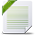# Power BI Exchange

View Only

## Product cost % expense to total cost#### Srikanth Hari11 days ago• #### 1.  Product cost % expense to total cost

Posted 11 days ago
Hi, Could you please help to derive the share (%) of expense to total cost, the % (category / total cost) is mentioned in the pbix but need a dax for the same

Thanks

------------------------------
FA
------------------------------

Attachment(s)% to total cost.pbix   24K 1 version

• #### 2.  RE: Product cost % expense to total cost

Posted 11 days ago
Hi,
Add this measure, it calculates percentage of each category in total.
Measure = DIVIDE(SUM('Table'[Cost]),CALCULATE(SUM('Table'[Cost]),ALL('Table'[Category])))------------------------------
Nemanja Andic
BI developer